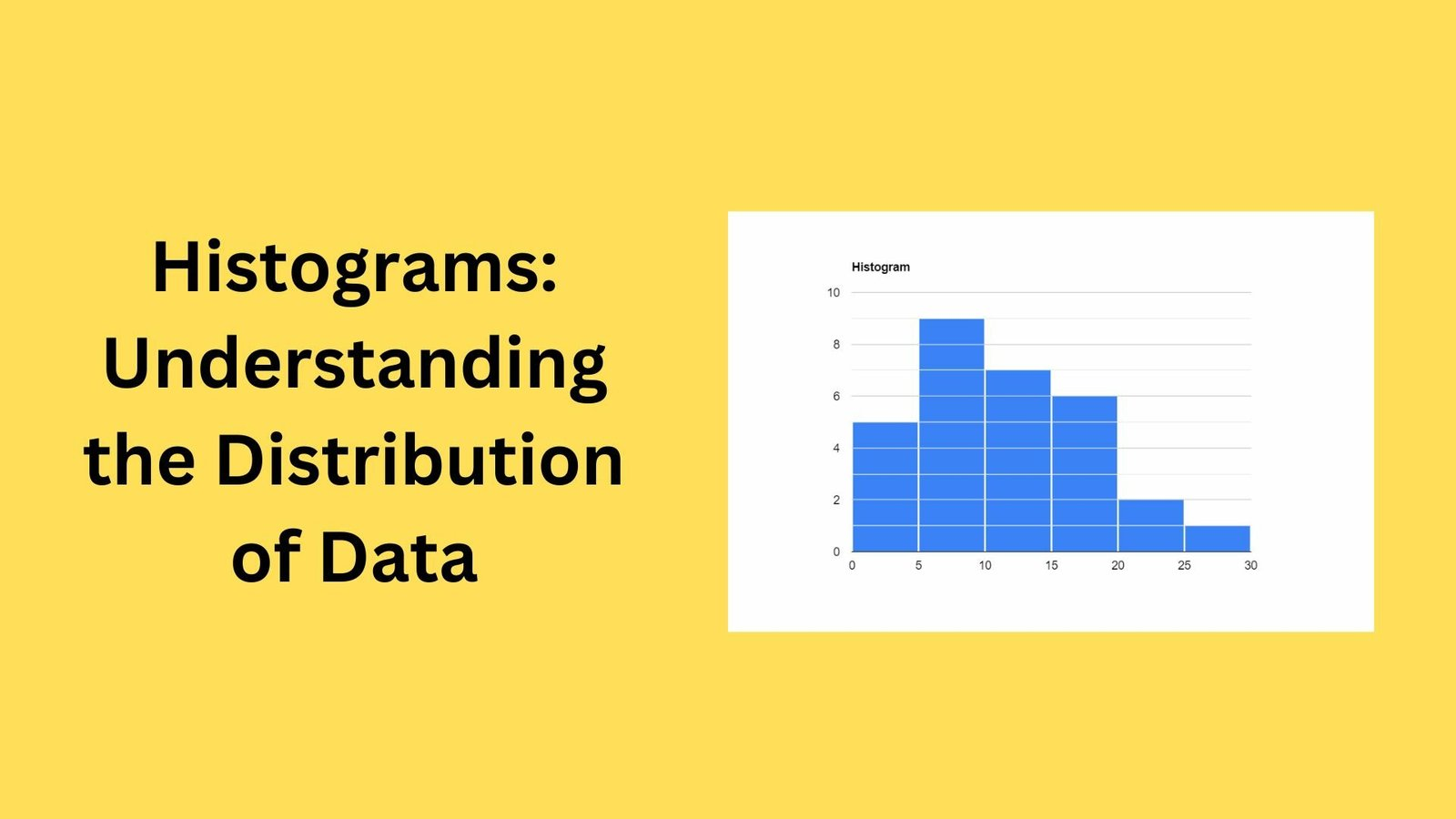# Histograms: Understanding the Distribution of DataHistograms are powerful tools in statistics that make this possible. Visualize the distribution of your dataset. you are special It helps to understand the distribution of continuous variables. weight, size, time, etc. This article describes it Defining, constructing, and interpreting histograms.

What is a Histogram?
A histogram is a graphical representation of data distribution. It's a way of showing the frequency of different values in a dataset. The X-axis represents the data values and the Y-axis represents the frequency of those values. Histograms typically consist of rectangular bars, with the height of each bar representing the frequency of values within that range.

Construction of Histograms
To create a histogram, the first step is to divide the range of values ​​into intervals (also called bins). The number of bins and the size of the intervals will depend on the specific data set and goals of the analysis. The next step is to count the number of observations that fall into each interval. This number is represented by the height of the rectangular bar in that interval.

Interpreting Histograms
A histogram is a visual representation of the distribution. record. It can be used to identify patterns, outliers, and skewness. in the data. For example, the symmetric distribution a A bell-shaped histogram, but with a skewed distribution Histogram with a long tail on one side. Additionally, the histogram It is used to identify outliers, i.e. values outside a range. The typical range of data.

Key Takeaways

• Histograms are powerful tools for visualizing the distribution of data.
• They are especially useful for understanding the distribution of continuous variables.
• Histograms are created by dividing a range of values into intervals and counting the number of observations that fall within each interval.
• You can use histograms to identify patterns, outliers, and skewness in your data.

Histograms are valuable tools for understanding the distribution of your data. Gain insight into patterns, outliers, and skewness in your data by creating and interpreting histograms. By applying this knowledge, you can make more informed decisions and gain a deeper understanding of your data.